Next: FILTERS IN PARALLEL Up: One-sided functions Previous: INVERSE FILTERS

# MINIMUM PHASE

In Sec. 2-1 we learned that knowledge of convergence of the Taylor series of 1/B(Z) on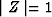is equivalent to knowledge that B(Z) has no roots inside the unit circle. Now we will see that these conditions are also equivalent to a certain behavior of the phase of B(Z) on the unit circle.

Let us consider the phase shift of the two-term filter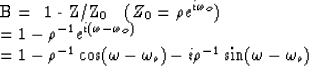By definition, phase is the arctangent of the ratio of the imaginary part to the real part.

A graph of phase as a function of frequency looks radically different for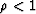than for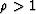. See Figure 4 for the case.

 2-4 Figure 4 Real and imaginary parts of the Z transform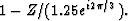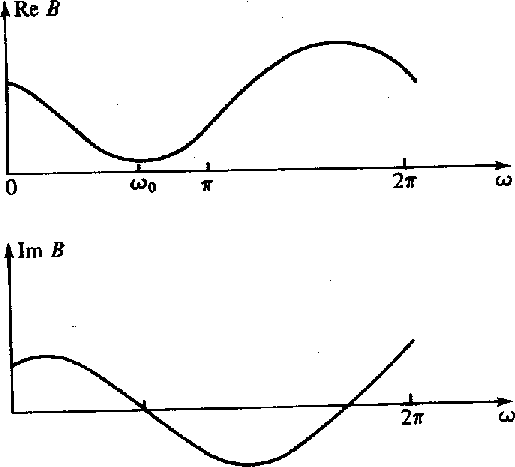The phase is the arctangent of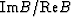. The easiest way to keep track of the phase is in the complex B plane. This is shown in Figure 5.

 2-5 Figure 5 Phase of the two-term filter of Figure 4.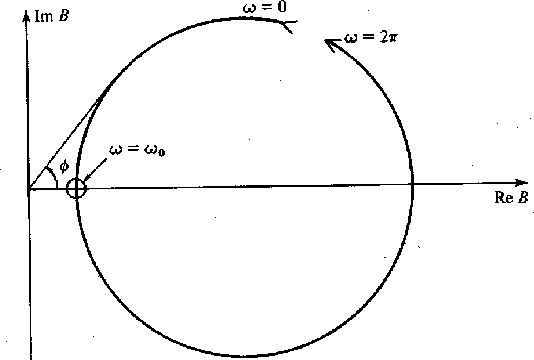Thus phase as a function of frequency is shown in Figure 6. Notice that the phase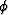at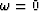is the same as the phase at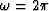. This follows because the real and imaginary parts are periodic with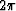. The situation will be different when there is a zero inside the unit circle; that is,. The real and imaginary parts are shown in Figure 7 and the complex plane in Figure 8.

 2-6 Figure 6 The phase of a two-term minimum-phase filter.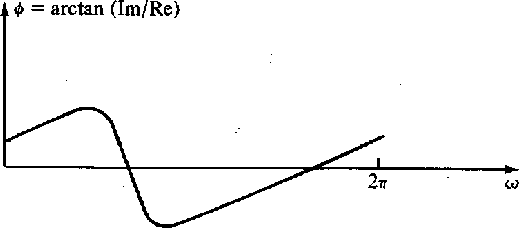2-7 Figure 7 Real and imaginary parts of the two-term nonminimum-phase filter,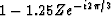.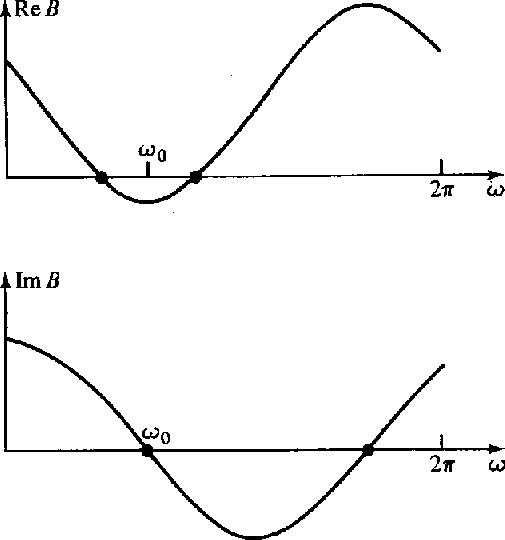2-8 Figure 8 Phase in complex plane.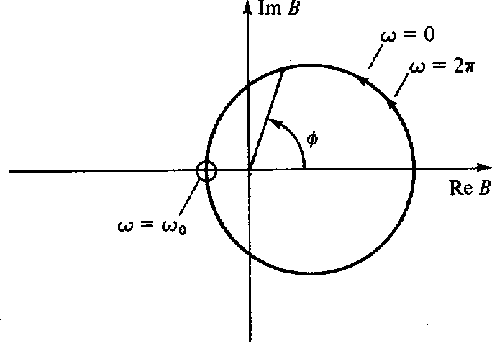The phaseincreases byas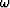goes from zero tobecause the circular path surrounds the origin. The phase curve is shown in Figure 9. The casewhere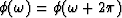has come to be called minimum phase or minimum delay.

 2-9 Figure 9 The phase of a two-term nonminimum-phase filter.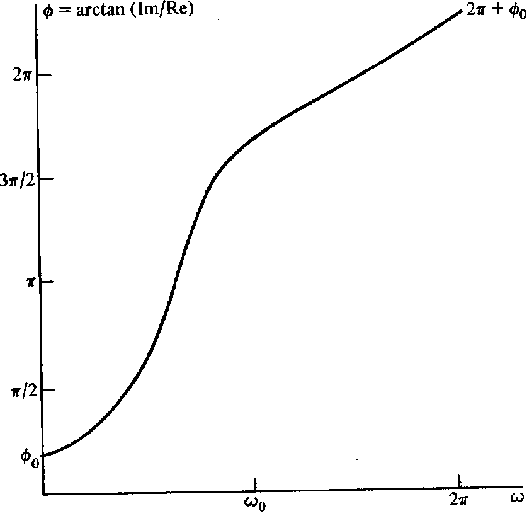Now we are ready to consider a complicated filter like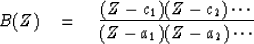(12)

By the rules of complex-number multiplication the phase of B(Z) is the sum of the phases in the numerator minus the sum of the phases in the denominator. Since we are discussing realizable filters the denominator factors must all be minimum phase, and so the denominator phase curve is a sum of curves like Figure 6. The numerator factors may or may not be minimum phase. Thus the numerator phase curve is a sum of curves like either Figure 6 or Figure 9. If any factors at all are like Figure 9, then the total phase will resemble Figure 9 in that the phase atwill be greater than the phase at. Then the filter will be nonminimum phase.Next: FILTERS IN PARALLEL Up: One-sided functions Previous: INVERSE FILTERS
Stanford Exploration Project
10/30/1997